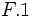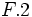# Free group:F2

View a complete list of particular groups (this is a very huge list!)[SHOW MORE]

## Definition

The free group of rank two, also written as$F_2$, is defined as the free group on a generating set of size two.$2$ is the smallest possible rank for a free non-abelian group (the free groups of rank$0$ and$1$ are respectively the trivial group and the group of integers).

The free group of rank two is a SQ-universal group. In particular, it has subgroups that are free of every finite rank as well as a free subgroup of countable rank.

## Arithmetic functions

Function Value Explanation
order infinite (countable)
exponent infinite (countable)
Fitting length not defined There is no nontrivial nilpotent normal subgroup.

## Group properties

Property Satisfied Explanation Comment
cyclic group No
abelian group No
nilpotent group No
solvable group No
free group Yes
hypocentral group Yes
hypoabelian group Yes
imperfect group Yes
finitely generated group Yes
slender group No There are free subgroups of countable rank.
centerless group Yes
Hopfian group Yes finitely generated and free implies Hopfian
co-Hopfian group No

## GAP implementation

The free group of rank two can be constructed using GAP with the GAP:FreeGroup command:

`FreeGroup(2);`

Further, the generators can also be referred to. For instance, if we use:

`F := FreeGroup(2);`

Then the two generators can be referred to as$F.1$ and$F.2$.